> restart;

>

Parametric Curves and Surfaces

>

Parametric Curves in 3D

Maple can be of great help plotting and visualizing parametric curves and surfaces. Consider a parametric curve in the three-dimensional space given by,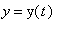,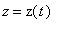,

where the parameter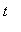is changing in some interval [a,b]. The proper command for plotting parametric curves is spacecurve that we used before. The command is contained in the plots package. Let's load the package and look at an example.

> with(plots):

>

Example 1. Consider an object moving in the xyz-space according to the equations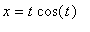,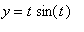,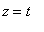,

where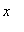,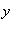,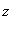are measured in feet,in minutes.

(a) Plot the object's path for t between 0 and 20.

(b) Find the distance traveled by the object during that time.

>

Let's define the coordinates x, y, z as expressions in terms of t and then plot the path.

> x:=t*cos(t); y:=t*sin(t); z:=t;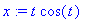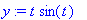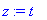Observe that the coordinates x, y, z under the spacecurve command (or formulas for those coordinates) are entered as a list, between square brackets.

> spacecurve([x,y,z],t=0..20,axes=normal,color=red,orientation=[54,64],labels=["x","y","z"]);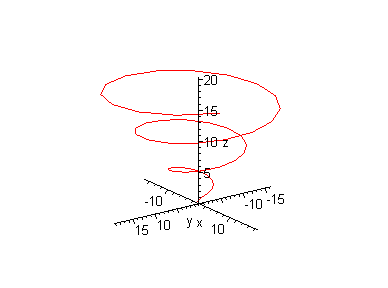>

Rotate the above graph to get a good sense of the motion. It's a kind of circular motion around the vertical axis with the z-coordinate and the radius increasing.

To calculate the distance traveled we have to evaluate the integral of the speed between t=0 and t=20. We use the numerical integration right away as we suspect the resulting integral is going to be too difficult to evaluate otherwise.

> Int(sqrt((diff(x,t)^2+diff(y,t)^2+diff(z,t)^2)),t=0..20); evalf(%);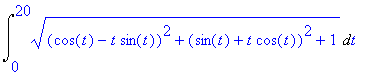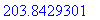>

The object has traveled almost 204 feet during the first twenty minutes.

After you are finished with a particular parametric representation, it is very important to restore the variables x, y, z, to being just variables again . It is accomplished by using the following commands:

> x:='x'; y:='y'; z:='z';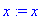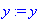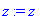>

Problem 1. An object is moving in the xyz-space along the trajectory defined by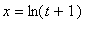,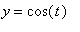,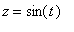,

where x, y, z are measured in meters, t in minutes.

(a) Plot the object's path for t between 0 and 25. Describe the motion in words.

(b) Calculate the distance traveled by the object during the 25 seconds.

Important Reminder ! If you assign formulas to x, y, z do not forget to unassign them by using the commands x:='x', y:='y', z:='z'.

>

Parmetric Surfaces in 3D

A parametric surface in xyz-space is, in general, given by the set of equations,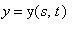,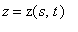,

where s, t are parameters with specified ranges. Of course, the parameters may be denoted by letters other than s and t. The basic syntax for plotting such surfaces uses the plot3d command and looks as in the following example.

Example 2. Plot the surface given by the parametric equations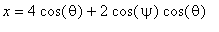,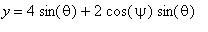,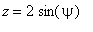,

where the parameters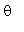and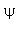both change between 0 and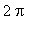.

We don't always have to define x, y, z, as expressions in terms of the parameters before plotting a curve or a surface. We can enter the formulas for x, y, z directly under the plot3d command as shown below. Observe that the formulas for the x, y, z coordinates are entered as a list between square brackets. Unless you tell Maple otherwise, it assumes that the three expressions between the brackets are formulas for the Cartesian coordinates x, y, z.

Greek letters are often used to denote parameters if the parameters have a geometric meaning of angles. Greek letters are entered using their full names. If you don't like it, you can always relabel the parameters. For example, to s and t.

> plot3d([4*cos(theta)+2*cos(psi)*cos(theta),4*sin(theta)+2*cos(psi)*sin(theta),2*sin(psi)],psi=0..2*Pi,theta=0..2*Pi,axes=framed,labels=["x","y","z"],scaling=constrained);>

Rotate the graph to get a good sense of the surface. The surface, called a torus , is a "doughnut" obtained by revolving a smaller circle, in our example of radius 2, along a bigger circle, in our example of radius 4. Try to see how the parameteris used to parametrize the big circle in the xy-plane, whileis used to parametrize smaller circles with the centers at each point of the larger circle. The scaling=constrained option allows us to see clearly the circular elements of the surface.

Problem 2 . Plot the surface given by the same parametric equations as in Example 2, but with the ranges for the parameters changed as follows:

(a)between 0 and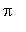,between 0 and. (b)between 0 andandbetween 0 and.

Rotate the graph obtained in (b) to see clearly the effects of the change in the range on the surface. Are the resulting graphs what you expected?

>

Example 3. Find a parametric representation of the surface>

We begin by rotating the surface to get a good sense of its shape. It seems that the suface is composed of circles with the center on the z-axis whose radius is changing as z is increasing from 0 to about 6. (Observe that the length of the unit on the x-axis and y-axis seems the same, so we do, indeed, have circles in planes parallel to the xy-plane.) For z=0, the radius is 1, then it decreases to 0 at about z=1.5, then increases again to 1. It seems that the radius is changing like cos(z). Let's try, then, the following parametrization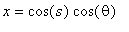,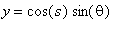,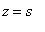,

for s andbetween 0 and. The first two coordinates describe the circle with radius cos(s) in the planeparallel to the xy-plane. The radius changes like cos(z). It should work. Let's have Maple plot our parametric surface.

> plot3d([cos(s)*cos(theta),cos(s)*sin(theta),s],theta=0..2*Pi,s=0..2*Pi,axes=framed,labels=["x","y","z"],orientation=[52,76]);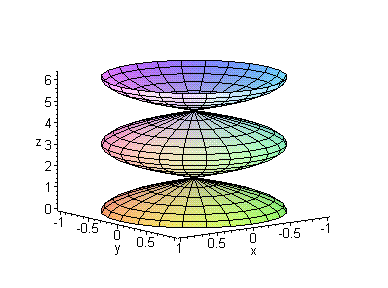>

It seems that our guess was correct!

>

Problem 3. Find a parametric representation of the following surfaces. Use Maple to check your answers.

(a)>

(b)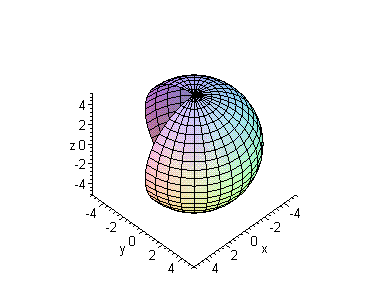>

(c)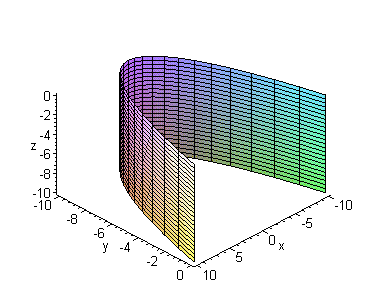>

Homework Problems

Your homework problems consist of Problems 1-3 above.

This MTH 243 Maple Worksheet written by D. Abrahamson, B. Kaskosz, and L. Pakula, Copyright 1999.

>